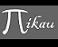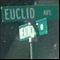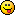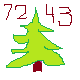Discussion about math, puzzles, games and fun.   Useful symbols: ÷ × ½ √ ∞ ≠ ≤ ≥ ≈ ⇒ ± ∈ Δ θ ∴ ∑ ∫  π  -¹ ² ³ °

You are not logged in.

## #1 2006-01-31 07:43:27

mikau
MemberRegistered: 2005-08-22
Posts: 1,504

### what is discrete math?

hope I spelled that right. Heard you take it in 3d game programming.

Also boolean algebra. Whats that about? In C++, type "bool" is a variable that only returns true or false. It does not hold a value.

A logarithm is just a misspelled algorithm.

Offline

## #2 2006-01-31 10:26:18

Ricky
ModeratorRegistered: 2005-12-04
Posts: 3,791

### Re: what is discrete math?

Discrete math is a compilation of different types of math.  My discrete math started off with something like boolean algebra.

Boolean algebra allows you to reduce statements into simpler ones.  For example:

Let a, b, and c be boolean.

(a and b) or (a and c) = a or (b and c), i.e. these two statements have the same truth values for any a, b, or c.  If (a and b) or (a and c) is false, so is a or (b and c).

It also has rules for negations:

not (a and b) = (not a) or (not b)

In programming, it makes writing if statements much more clear.

Discrete math, for me, went from boolean algebra, to proofs (forward, contrapositive, contradiction, and induction), to sets, operations, and functions.

The only application for discrete math in programming is function analysis, i.e. seeing how long a piece of code would take to run.

"In the real world, this would be a problem.  But in mathematics, we can just define a place where this problem doesn't exist.  So we'll go ahead and do that now..."

Offline

## #3 2006-01-31 18:32:16

mikau
MemberRegistered: 2005-08-22
Posts: 1,504

### Re: what is discrete math?

Interesting. Thanks for the description.

A logarithm is just a misspelled algorithm.

Offline

## #4 2006-01-31 20:25:25

MathsIsFun
AdministratorRegistered: 2005-01-21
Posts: 7,694

### Re: what is discrete math?

mikau wrote:

It does not hold a value.

Ah, but it does! It holds a binary value, the basis of life as we know it.

"The physicists defer only to mathematicians, and the mathematicians defer only to God ..."  - Leon M. Lederman

Offline

## #5 2006-02-01 09:37:16

Ricky
ModeratorRegistered: 2005-12-04
Posts: 3,791

### Re: what is discrete math?

In C++, type "bool" is a variable that only returns true or false. It does not hold a value.

Little known fact, most compilers have a bool as 8 bits, even though only one is needed.

"In the real world, this would be a problem.  But in mathematics, we can just define a place where this problem doesn't exist.  So we'll go ahead and do that now..."

Offline

## #6 2006-02-01 09:58:17

mikau
MemberRegistered: 2005-08-22
Posts: 1,504

### Re: what is discrete math?

Yeah I meant it doesn't store a numerical value for the user.

Why the heck is it 8 bits on some compilers? I thought the purpose was to save memory for switch variables. Peculiar...

A logarithm is just a misspelled algorithm.

Offline

## #7 2006-02-01 10:31:13

ryos
MemberRegistered: 2005-08-04
Posts: 394

### Re: what is discrete math?

Does boolean algebra have a distributive property?

For example, I often wish I could say: a == (b or c or d)
instead of: (a == b) or (a == c) or (a == d)
...but the first form doesn't work.

El que pega primero pega dos veces.

Offline

## #8 2006-02-01 10:34:37

mikau
MemberRegistered: 2005-08-22
Posts: 1,504

### Re: what is discrete math?

like in C++?

Last edited by mikau (2006-02-01 10:34:52)

A logarithm is just a misspelled algorithm.

Offline

## #9 2006-02-01 10:43:01

ryos
MemberRegistered: 2005-08-04
Posts: 394

### Re: what is discrete math?

Well, PHP. I've never written a line of C++, and hope I never have to.

El que pega primero pega dos veces.

Offline

## #10 2006-02-01 10:46:21

mikau
MemberRegistered: 2005-08-22
Posts: 1,504

### Re: what is discrete math?

Its not that hard to use once you know it. But it can very hard to learn sometimes.

A logarithm is just a misspelled algorithm.

Offline

## #11 2006-02-01 10:56:08

Ricky
ModeratorRegistered: 2005-12-04
Posts: 3,791

### Re: what is discrete math?

Does boolean algebra have a distributive property?

For example, I often wish I could say: a == (b or c or d)
instead of: (a == b) or (a == c) or (a == d)
...but the first form doesn't work.

Not quite.  There is no equality in boolean algebra.  Instead, each variable is either true or false.

So for your example, if you had:

a and (b or c or d)

the equivalent would be:

(a and b) or (a and c) or (a and d)

"In the real world, this would be a problem.  But in mathematics, we can just define a place where this problem doesn't exist.  So we'll go ahead and do that now..."

Offline

## #12 2006-02-01 10:59:13

Ricky
ModeratorRegistered: 2005-12-04
Posts: 3,791

### Re: what is discrete math?

Why the heck is it 8 bits on some compilers? I thought the purpose was to save memory for switch variables. Peculiar...

This is a bit of a complex question (pun intended).  Computers run much faster if all data is stored in whole bytes.  To understand why, you need to understand the underlying architecture, which would take me a while to describe.

"In the real world, this would be a problem.  But in mathematics, we can just define a place where this problem doesn't exist.  So we'll go ahead and do that now..."

Offline

## #13 2006-02-01 11:00:45

ryos
MemberRegistered: 2005-08-04
Posts: 394

### Re: what is discrete math?

Heh, I think exactly opposite. My first programming language was C at age 13. My first OOP language was Java at age 18. Both were very easy to learn, but using Java is much easier. This is mainly due to Java's memory handling and extensive API. And after using a language that abstracts strings, I just can't bring myself to use a language that doesn't (of course, I'm sure people have abstracted strings tons of times in C++, but I doubt it's as slick as native support).

I'm starting to learn Ruby, which is supposedly nice enough to make PHP blush with shame.

El que pega primero pega dos veces.

Offline

## #14 2006-02-01 11:13:23

mikau
MemberRegistered: 2005-08-22
Posts: 1,504

### Re: what is discrete math?

Well in the case of C++, there are I believe three logical operators,  And "&&" Or, "||" and Not "!"

Really an if statement returns true if the statement is true, and false if the statement is false, but you can use simple values instead of relational opererators such as ==, >, <, etc. A variable with a value of  zero evaluates false. All others evaluate true.

If for instance you were to say:

if(5) { statement; }

it will evaluate true and always will since 5 does not equal zero, and the statement will be excecuted.

if(0) { statement; }

this will not be executed. Zero returns false.

When you use the logical operators,  you can use the "or" and "and" operaters to execute a statement if either, or only if both are true.

you could say

if(0 || 1) { statement; }

that would return true. ( "||" means or)

if (0 && 1) { statement; }

that would not return true since both must be true.

But when you say if ( x == (5 || 7)) { statement;}

I believe this is incorrect use of the or operator. The or operator is used between two expressions that are evaluated for their truth or falsity. Not their value.

A logarithm is just a misspelled algorithm.

Offline

## #15 2006-02-02 06:08:46

ryos
MemberRegistered: 2005-08-04
Posts: 394

### Re: what is discrete math?

OK. Too right.I still wish their were a language construct for compacting conditions wherein a variable could equal one of several things that trigger the condition.

El que pega primero pega dos veces.

Offline

## #16 2006-02-02 06:22:04

mikau
MemberRegistered: 2005-08-22
Posts: 1,504

### Re: what is discrete math?

maybe there is. Many computer languages hold secret techniques only known by the ninja's.

A logarithm is just a misspelled algorithm.

Offline

## #17 2006-02-02 08:29:17

MathsIsFun
AdministratorRegistered: 2005-01-21
Posts: 7,694

### Re: what is discrete math?

ryos wrote:

OK. Too right.I still wish their were a language construct for compacting conditions wherein a variable could equal one of several things that trigger the condition.

Isn't that the "select" statement (aso called "case" or "switch") ?

Example (Visual Basic code to replace any non-alphanumeric with "_"):

``````    Select Case c
Case "a" To "z", "A" To "Z", "0" To "9"
Mid\$(x, i, 1) = c

Case Else
Mid\$(x, i, 1) = "_"

End Select``````

I chose VB because it has such a rich case statement, PHP can only have one value per "case":

``````    switch (\$MatchType) {
case "=":
\$s .= \$MatchVar . "=" . \$MatchVal;
break;

case "Like":
\$s .= \$MatchVar . ' like "%' . \$MatchVal . '%"';
break;

default:
\$s .= \$MatchVar . \$MatchVal; break;

}``````

But PHP, like C, will continue to run statements below it without the "break;", so this can be used to put several "case" statments in sequence:

``````    switch (\$MatchType) {
case "=":
case "equal":
case "same":
\$s .= \$MatchVar . "=" . \$MatchVal;
break;

case "Like":
\$s .= \$MatchVar . ' like "%' . \$MatchVal . '%"';
break;

default:
\$s .= \$MatchVar . \$MatchVal; break;

}``````

But there is a trick in PHP: use switch (TRUE) and then the "case"s can be conditions like "(\$x >5)" or "in_array()" etc.

Sorry, didn't mean to ramble on.

"The physicists defer only to mathematicians, and the mathematicians defer only to God ..."  - Leon M. Lederman

Offline

## #18 2006-02-02 11:26:30

ryos
MemberRegistered: 2005-08-04
Posts: 394

### Re: what is discrete math?

Hmm...case true eh? Pretty slick. I had thought of the rollover switch, but it seems like using a sledgehammer to open a can. Almost easier to just use a knife. But what I really need is a can opener...

El que pega primero pega dos veces.

Offline

## #19 2006-02-02 20:13:02

MathsIsFun
AdministratorRegistered: 2005-01-21
Posts: 7,694

### Re: what is discrete math?

``````canopener (\$problem) {
\$result = solved;
}``````

"The physicists defer only to mathematicians, and the mathematicians defer only to God ..."  - Leon M. Lederman

Offline

## #20 2006-02-03 09:03:26

ryos
MemberRegistered: 2005-08-04
Posts: 394

### Re: what is discrete math?

``````<?php
function canOpener (\$can)  {
\$canOpened = false;
\$args = func_get_args();
unset (\$args);  //that's \$can; we have that one

foreach (\$args as \$compareTo)  {
if (\$can == \$compareTo)  { \$canOpened = true; }
}

return \$canOpened;
}
?>``````El que pega primero pega dos veces.

Offline

## #21 2006-02-04 03:24:29

John E. Franklin
MemberRegistered: 2005-08-29
Posts: 3,588

### Re: what is discrete math?

Also on the topic of discrete math for electronics is Karnaugh mapping created in 1950's.
And Logic Circuit Minimization.

igloo myrtilles fourmis

Offline

## #22 2019-07-21 07:57:43

John E. Franklin(2)
MemberRegistered: 2019-05-17
Posts: 17

### Re: what is discrete math?

00 = 0 trivial case, FALSE

01 = 33 & 05, where 05 = 55 & 0F

03 = 33 & 0F

09 = 99 & 0F, where 99 = CC XOR 55

81 = C3 & A5, where C3 = F0 XOR 33, and where A5 = F0 XOR 55

07 = 0F & 77, where 77 = 55 OR 33

83 = F0 XOR 73, where 73 = 50 OR 33, where 50 = F0 & 55

49 = AA XOR E3, where E3 = C3 OR A0, and where C3 = F0 XOR 33, and A0 = F0 & AA

0F = trivial case, a boolean variable.

96 = CC XOR 5A, where 5A = F0 XOR AA

35 = C0 XOR F5, where C0 = F0 & CC and where F5 = F0 OR 55

39 = CC XOR F5, where F5 = F0 OR 55

17 = C0 XOR D7, where C0 = F0 & CC and where D7 = C3 OR 55, and where C3 = F0 XOR 33

99 = CC XOR 55

These are 3-variable cases above.
Use a cube by folding the 2 by 4 Karnaugh map in half.
There are 22 shapes for 3 variables.

Offline

## #23 2019-07-22 17:25:54

John E. Franklin(2)
MemberRegistered: 2019-05-17
Posts: 17

### Re: what is discrete math?

``````0 2 6 4
1 3 7 5

2**0 = 1  2**2=4  2**6=64=40Hex  2**4=16=10Hex
2**1 = 2  2**3=8  2**7=128=80Hex 2**5=32=20Hex

01 04 40 10
02 08 80 20

Add these numbers up.

FF = tautology, always true
00 = falsehood, contradiciton, always false.
F0 = A
0F = not A = /A
55 = B
AA = not B = /B
CC = C
33 = not C = /C
...Note A, B, and C are interchangeable to your liking when starting the definitions...(it depends on the definition of your axes.)
The variables A, B, and C are inputs that create the output conditions when combined together with AND, OR, and XOR,
and also IMP and others...``````

Offline

## #24 2019-08-13 12:22:09

John E. Franklin(2)
MemberRegistered: 2019-05-17
Posts: 17

### Re: what is discrete math?

...So for 3 variables, the 3 circles of a Venn diagram may end up as the front, top, and left of a cube.
The 8 corners of a cube designate the 8 areas in a Venn diagram.  You must count the outside area
as the eighth area in a Venn diagram with 3 circles.  Now if you have this cube with 8 corners, the
corners can have colored beads on the corners.  Since this is boolean algebra, and not ternary, there
are 2 colors (boolean = 2 values, black and white, orange and pear, etc.)  Now the input variables
are usually A, B, and C.    The colors of the corners is the output of true or false (boolean, true or false),
such as the outputs on a "truth table".  Now if you choose the left or right side of the cube, that could
be the variable A.  (Any way you choose is okay, left/right, top/bottom, front/back)  Now the B
variable could be the top and the negation of B would then be the bottom of the cube, 4 corners on
the bottom.  Finally the C variable could the front 4 corners of the cube and the negation of C (called
"C not") could be the back 4 corners of the cube.  Now the fun begins if you combine the different
halves of the cube together with boolean operators such as NAND, AND, OR, NOR, XOR, EQUALITYGATE,
IMPLICATIONGATE, etc....  When you combine two halves of the cube together, then you get a
different shape or pattern of black and white beads in the corners.  What I did is write a short
computer program to iterate all possible combinations of operators and keep track of the complexity
of the equation, by counting the operators in the equation.  Operators is called "gates" in digital
electronics.  I ran the 3 variable case on a PC, and it runs in under a second.  Then I changed
the program (written in C) to 4 variable case (hypercube) and that runs in 20 minutes.  The
result is an equation for all 65536 four variable cases...  Anyway, it was a lot of the fun.
That's all for now, maybe I'll write more later on this in a couple months... Bye.

Offline

## Board footer

Powered by FluxBB